## Exterior Derivative

Consider a Differential k-Form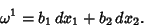(1)

Then its exterior derivative is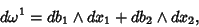(2)

where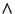is the Wedge Product. Similarly, consider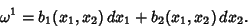(3)

Then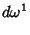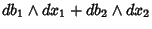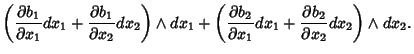(4)

Denote the exterior derivative by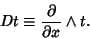(5)

Then for a 0-form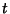,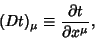(6)

for a 1-form,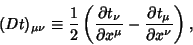(7)

and for a 2-form,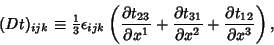(8)

where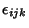is the Permutation Tensor.

The second exterior derivative is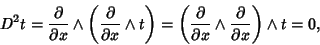(9)

which is known as Poincaré's Lemma.